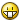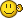## Recommended Posts

hello world!

i have strings that vary positioning for superfluous commas - sometimes extra commas in the middle, beginning or end of the string

this is the method im using to clean up the string - but i dont think its the cleanest solution

any ideas?  stringregexp perhaps?

```#include <Array.au3>
\$msg_normal = 262144

\$string = ",,,,asdf,as32,,,3k2,as12,"

\$array = StringSplit(\$string, ",")

debug(\$array)

\$new_string = ""

for \$x = 1 to UBound(\$array)-1
if \$array[\$x] = "" then ContinueLoop

\$new_string &= \$array[\$x] & ","
Next

\$new_string = StringTrimRight(\$new_string, 1)

debug(\$new_string)

Func Debug(\$variable1 = "", \$variable2 = "", \$variable3 = "")

;~  #include <array.au3>
;~  \$msg_normal = 0

If IsArray(\$variable1) Then
_ArrayDisplay(\$variable1)
Else
If \$variable2 <> "" Then
\$variable1 &= @CRLF & \$variable2
EndIf

If \$variable3 <> "" Then
\$variable1 &= @CRLF & \$variable3
EndIf

ClipPut(\$variable1)
MsgBox(\$msg_normal, "Debug", \$variable1)
EndIf

EndFunc   ;==>Debug```

##### Share on other sites

What's your final output expected to be?  If this "asdf,as32,3k2,as12", then

```\$string = ",,,,asdf,as32,,,3k2,as12,"
\$string2 = StringRegExpReplace(\$string,",+",",")
\$string2 = StringRegExpReplace(\$string2,"\B,|,\B","")
ConsoleWrite(\$string2 & @CRLF)```
IEbyXPATH-Grab IE DOM objects by XPATH IEscriptRecord-Makings of an IE script recorder ExcelFromXML-Create Excel docs without excel installed GetAllWindowControls-Output all control data on a given window.
##### Share on other sites

it is - sorry should have mentioned thatthank you very much!!

Edited by gcue
##### Share on other sites

what if the character was | instead of comma?  i tried replacing the character but doesnt work..

##### Share on other sites

You would need to know how to backtrack in the search, which I don't, so I didn't.  If you could find a way to find all three situations, and replace with the exact same result, then you could do a simple regexpreplace.

IEbyXPATH-Grab IE DOM objects by XPATH IEscriptRecord-Makings of an IE script recorder ExcelFromXML-Create Excel docs without excel installed GetAllWindowControls-Output all control data on a given window.
##### Share on other sites

hmm i dont either -  i think i may just resort back to my original method

```Func StripSuperfluousCharacter(\$string, \$character)

\$array = StringSplit(\$string, \$character)

\$new_string = ""

for \$x = 1 to UBound(\$array)-1
if \$array[\$x] = "" then ContinueLoop

\$new_string &= \$array[\$x] & \$character
Next

\$new_string = StringTrimRight(\$new_string, 1)

return \$new_string

EndFun```

thanks again for your help =)

Edited by gcue
##### Share on other sites

```#include <Array.au3>

\$string = ",,,,asdf,as32,,,3k2,as12,"
\$delimeter = ","
\$aString = stringsplit(\$string , "" , 3)

If \$aString[ubound(\$aString) - 1] = \$delimeter then _ArrayPop(\$aString)

For \$i = ubound(\$aString) - 1 to 1 step -1
If \$aString[\$i] = \$delimeter AND \$aString[\$i - 1] = \$delimeter Then _ArrayDelete(\$aString , \$i)
Next

If \$aString = \$delimeter then _ArrayDelete(\$aString , 0)

msgbox(0 , '' , _ArrayToString(\$aString , ""))```

Edited by boththose

```,-. .--. ________ .-. .-. ,---. ,-. .-. .-. .-.
|(| / /\ \ |\ /| |__ __||| | | || .-' | |/ / \ \_/ )/
(_) / /__\ \ |(\ / | )| | | `-' | | `-. | | / __ \ (_)
| | | __ | (_)\/ | (_) | | .-. | | .-' | | \ |__| ) (
| | | | |)| | \ / | | | | | |)| | `--. | |) \ | |
`-' |_| (_) | |\/| | `-' /( (_)/( __.' |((_)-' /(_|
'-' '-' (__) (__) (_) (__)```

##### Share on other sites

A regex way :

```; \$string = ",,,,asdf,as32,,,3k2,as12,"
; \$delimeter  = ","

\$string = "||||asdf|as32|||3k2|as12|"
\$delimeter  = "|"

\$string2 = StringRegExpReplace(\$string, "(\Q" & \$delimeter & "\E)(?=(?1)|\$)","")
ConsoleWrite(\$string2 & @CRLF)```

##### Share on other sites

A regex way :

```; \$string = ",,,,asdf,as32,,,3k2,as12,"
; \$delimeter  = ","

\$string = "||||asdf|as32|||3k2|as12|"
\$delimeter  = "|"

\$string2 = StringRegExpReplace(\$string, "(\Q" & \$delimeter & "\E)(?=(?1)|\$)","")
ConsoleWrite(\$string2 & @CRLF)```

works great... except it doesnt remove the leading instance of the delimiter

```\$string = "||||asdf|||||||||||||||||||||||||||||||||||||||||||||||||||||||as32|3k2|as12||||||||"
\$delimeter  = "|"

\$string2 = StringRegExpReplace(\$string, "(\Q" & \$delimeter & "\E)(?=(?1)|\$)","")

debug(\$string2)```
##### Share on other sites

so add one more line?  JG, that is a nice regex btw.

```\$string2 = StringRegExpReplace(\$string, "(\Q" & \$delimeter & "\E)(?=(?1)|\$)","")
If stringleft(\$string2 , 1) = \$delimeter Then \$string2 = stringtrimleft(\$string2, 1)

msgbox(0 , '' , \$string2)```

```,-. .--. ________ .-. .-. ,---. ,-. .-. .-. .-.
|(| / /\ \ |\ /| |__ __||| | | || .-' | |/ / \ \_/ )/
(_) / /__\ \ |(\ / | )| | | `-' | | `-. | | / __ \ (_)
| | | __ | (_)\/ | (_) | | .-. | | .-' | | \ |__| ) (
| | | | |)| | \ / | | | | | |)| | `--. | |) \ | |
`-' |_| (_) | |\/| | `-' /( (_)/( __.' |((_)-' /(_|
'-' '-' (__) (__) (_) (__)```

##### Share on other sites

`\$string2 = StringRegExpReplace(\$string, "^(\Q" & \$delimeter & "\E)*|(?1)(?=(?1)|\$)","")`Edited by mikell
##### Share on other sites

Try this one :

`\$string2 = StringRegExpReplace(\$string, "^(\Q" & \$delimeter & "\E)+|(?1)(?=(?1))|(?1)+\$","")`

Edit : oops, Mikell made the compact version...Edit2 : thanks boththose !

Edited by jguinch

##### Share on other sites

Yo my friendTwas just a slight modification of yours...

## Create an account

Register a new account

• ### Recently Browsing   0 members

×

• Wiki

• Back

• #### Beta

• Git
• FAQ
×
• Create New...